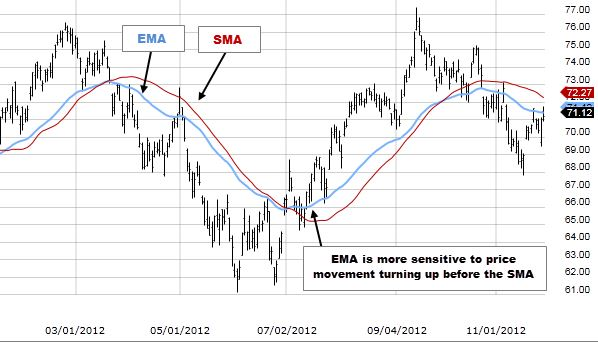## How to use the EMA forex trading strategy?

Jan 15 • Forex Trading Articles • 1325 Views • Comments Off on How to use the EMA forex trading strategy?

Exponential moving average, or the EMA, is a technical indicator that differs from other moving averages. Its calculations give greater weight to the most recent price data. Therefore, the EMA offers importance to the most recent behavior of traders.

It means that the exponential moving average indicator can react at a faster rate to changes in the price of an asset.

It does not limit using EMA as part of your trading strategy to a specific instrument. It is one way in which you can set up an EMA line for a variety of trading instruments.

The simplest method is the Simple Moving Average (SMA). This method considers the price values equally. After this, the next step is to take the mean of the average.

The other common types of moving average assign a weighting to different price values, favoring recent prices more heavily than older prices.

## How to Calculate the Exponential Moving Average?

We calculate an EMA at the time – t – using the exponential moving average formula as follows:

EMAt = α x current price + (1- α) x EMAt-1

It is quite evident from the initial formula that calculating the EMA for a given point in time requires that we know the EMA from the previous times.

So the traders derive the current value of the last day’s EMA for a daily EMA. Then, the traders derive from the day before that, and the process goes on.

## How to Read the Exponential Moving Average?

If we talk about the exponential moving average strategy, the most commonly used time periods used by traders in setting an EMA frame are 50-, 100- and 200-day periods for the long-term line.

A typical short-term time frames used by traders are the 12-day and 26-day EMAs. It will help if you remember to change the EMA set-up when you trade new instruments because there is no one-size-fits-all structure for an EMA indicator.

## Exponential Moving Average Trading Strategy

There is an even more effective way of producing trading signals is using a double exponential moving average combination, one short-term and one long-term.

When the shorter EMA crosses, the longer one, the exponential moving average crossover strategy creates a trading signal.

An example of this would be if a long-term trend trader might use a 25-day EMA as the shorter average. Then he would use a 100-day EMA as the long-term trend line.

When there is such an exponential moving average strategy, the trader will buy when the 25-day EMA crosses above the 100-day EMA. They would then sell when the 25-day EMA crosses below the 100-day EMA.

## Bottom line

There are quite a few advantages of this trend. Not only does it confirm the movement, but it can also provide trading signals, as we can see in the exponential moving average crossover strategy. If we talk about it in amateur terms, an exponential moving average will respond quicker to newer data than an SMA, as it assigns more weight to more recent prices.